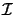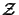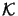Next: 6. Differential Method Up: 5.3 Field Calculation over Previous: 5.3.1 Rigorous Electromagnetic Solution

## 5.3.2 Approximate Electromagnetic Solution

All of the methods described so far calculate the EM field in a rigorous manner. As can be seen from Table 5.1 the computational requirements of these methods are extremely high. Especially in three-dimensions their application is often prohibitively expensive. Hence various computationally less expensive albeit approximate methods have been proposed recently. The first efforts were reported by Jörg Bischoff et al. [191,192], when he adapted the geometrical theory of diffraction  for scattering investigations in lithography. This theory is an extension to geometrical optics by introducing--in addition to the usual geometrical rays--diffracted ones that describe the influence of edges, corners, or vertices at the boundary surfaces. A similar approach was refined and extended by Michael Yeung and Andrew Neureuther and is commonly referred to as the physical-optics approximation [194,195]. According to diffraction theory, the field representing the light within a homogeneous volume is uniquely determined by the values of the tangential field components over the surface enclosing the volume (cf. the integral theorem in (4.5)). In the zeroth-order physical-optics approximation the incident plane wave induces a tangential electric and magnetic field at each point on the enclosing surface that would be present on the tangent plane at that point, i.e., by the sum of the incident and the reflected field of the tangent plane. Based on an accuracy examination performed by comparison with the results of a rigorous method, they introduced sophisticated corrections to non-physical simulation artifacts like edge currents . Additionally, special care has to be given to inter-surface as well as intra-surface multiple scattering in case of multilayer structures. A considerable speed-up can be achieved by grouping neighboring surface elements together and use generalized Sommerfeld integrals [196,197] to represent the vector multiple waves instead of treating each surface element separately as independent source of radiation . This acceleration technique is known as fast multipole method [198,199]. The resulting overall algorithm is restricted to piecewise linear topography but it is extremely fast in comparison to the rigorous methods (cf. Table 5.1). The fast multipole method can also be extended for use in an integral-equation approach suited for arbitrary topography. The expected performance is given in the last row of Table 5.1. Due to their moderate storage and computational costs approximate methods are a valuable alternative to the rigorous approaches although their application is physically limited.

Table 5.1: Comparison of rigorous and approximate simulation techniques of topography scattering in two and three dimensions with n as the number of grid points in each dimension (after ). Note that the statements on the computational costs are somehow misleading as the required grid density crucially depends on the respective approach. For example, the time-domain finite-difference method needs a much finer grid than the two frequency-domain methods of the waveguide and differential model. However, the information provided gives a direction onto the actual requirements.

 Method Features Storage CPU Availability Spectral-element Sparse matrix, direct solution n5 n3 n7 n4 (Philips/Signetics, 2D) Time-domain finite-difference Massively parallel n3 n2 n3 xn2 xTEMPEST (UC Berkeley, 2D&3D) Waveguide Small refractive index changes n4 n2 n6 xn3 xMETROPOLE (Carnegie-Mellon, 2D&3D) Differential Smooth topography n4 n2 n6 xn3 xiPHOTO (Intel, 2D) LISI (TU Vienna, 3D) Physical-optics & fast multipole Piecewise linear topography n2 n n3 n1.5 (UC Berkeley, 3D) Integral & fast multipole Iterative solution n2 n n3.3 xn1.5 x(UC Berkeley, 3D)

Upper quantities characterize the storage and computational costs for three-dimensional simulations, whereas lower quantities refer to two-dimensions. The symbolsandsignify the number of iterations required for the respective technique, anddenotes the accuracy of the vertical discretization.Next: 6. Differential Method Up: 5.3 Field Calculation over Previous: 5.3.1 Rigorous Electromagnetic Solution
Heinrich Kirchauer, Institute for Microelectronics, TU Vienna
1998-04-17